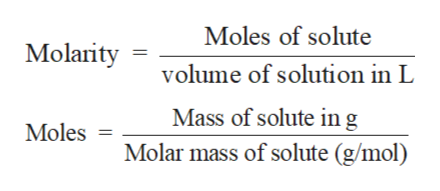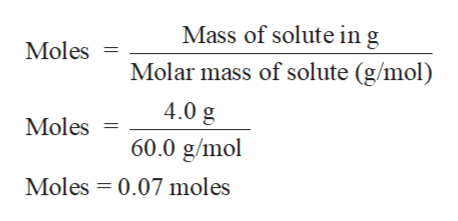# Vinegar contains (among other things) acetic acid. What is the molarity of acetic acid (HC2H3O2) in vinegar that contains 4.0% acetic acid by weight and having a density of 1.02 g/mL?

Question
8 views

Vinegar contains (among other things) acetic acid. What is the molarity of acetic acid (HC2H3O2) in vinegar that contains 4.0% acetic acid by weight and having a density of 1.02 g/mL?

check_circle

Step 1

Solution stoichiometry involves the calculation of concentration of solutions in the given conditions of volumes, moles etc.

Solution stoichiometry is mainly based on the calculation of moles and volumes. These two values are used to calculate the molarity of solution. The relation between moles, volume and molarity is as given below;help_outlineImage TranscriptioncloseMoles of solute Molarity volume of solution in L Mass of solute ing Moles Molar mass of solute (g/mol) fullscreen
Step 2

Mass percentage of acetic acid in vinegar = 4.0 %

Hence 4.0 g of acetic acid is present in 100 g of solution

Molar mass of acetic acid = 60 g/molhelp_outlineImage TranscriptioncloseMass of solute in g Moles Molar mass of solute (g/mol) 4.0 g Moles 60.0 g/mol Moles 0.07 moles fullscreen
Step 3

Density of solution = 1.02 g/mL

Mass of solution = 100 g (from 4.0 ...

### Want to see the full answer?

See Solution

#### Want to see this answer and more?

Solutions are written by subject experts who are available 24/7. Questions are typically answered within 1 hour.*

See Solution
*Response times may vary by subject and question.
Tagged in

### Solutions